new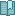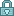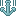Eric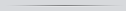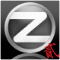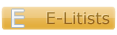1,442November 2005
 [Python] Tic-Tac-Toe Jun 23, 2009 11:05:37 GMT   I had to, haha`class Board: """A tic-tac-toe board.""" def __init__(self): self.squares = {} self.clear() def set_square(self, sq, piece): self.squares[sq] = piece; def clear_square(self, sq): self.squares[sq] = '-' def clear(self): for x in range(9): self.squares[x] = '-' def get_pieces(self, piece): opensq = [] for x,y in self.squares.items(): if y == piece: opensq.append(x) return set(opensq) def open_squares(self): return self.get_pieces("-") def render(self): for x in range(9): print(self.squares[x], end="") if x % 3 == 2: print()class Player: """A tic-tac-toe player""" def __init__(self, piece): self.piece = piece def make_move(self, game): passclass HumanPlayer(Player): def make_move(self, game): sq = "a" sqs = game.open_squares() print("Which square would you like (1-9)?") while sq not in sqs: try: sq = int(input("> "))-1 except: sq = "a" print("A number 1-9 please") game.set_square(sq, self.piece)class AIPlayer(Player): def choose_move(self, game, player, oplayer, alpha=-100, beta=100, first=False): if game.game_over(): return game.evaluate(player) best = -2 best_sq = -1 moves = game.open_squares() for move in moves: game.set_square(move, player.piece) alpha = max(alpha, -self.choose_move(game, oplayer, player, -beta, -alpha)) game.clear_square(move) if alpha > best: best = alpha best_sq = move if(alpha >= beta): break; if first: return best_sq return best def make_move(self, game): if game.player1 == self: y = game.player2 else: y = game.player1 game.set_square(self.choose_move(game, self, y, first=True), self.piece)class Game: def __init__(self, p1, p2): self.board = Board() self.player1 = p1 self.player2 = p2 self.turn = p1 self.wins = ({0,1,2},{3,4,5},{6,7,8},{0,3,6}, {1,4,7},{2,5,8},{0,4,8},{2,4,6}) def set_square(self, sq, piece): self.board.set_square(sq, piece) def clear_square(self, sq): self.board.clear_square(sq) def open_squares(self): return self.board.open_squares() def reset(self): self.board.clear() def check_win(self, player): psquares = self.board.get_pieces(player.piece) for win in self.wins: if psquares & win == win: return True return False def check_filled(self): if len(self.open_squares()) == 0: return True return False def game_over(self): if self.check_win(self.player1) or \ self.check_win(self.player2) or \ self.check_filled(): return True return False def evaluate(self, player): p1win = self.check_win(self.player1) p2win = self.check_win(self.player2) if p1win: if player == self.player1: return 1 return -1 if p2win: if player == self.player2: return 1 return -1 return 0 def play(self): while not self.game_over(): self.board.render() self.turn.make_move(self) if self.turn == self.player1: self.turn = self.player2 else: self.turn = self.player1 self.board.render() end = self.evaluate(self.player1) if end == 1: print("Player one wins!") elif end == -1: print("Player two wins!") else: print("Cat's game!")val = "x"print("Is player one human?")while val != "y" and val != "n": val = input("y/n: ").lower()if val == "y": h = HumanPlayer("X")else: h = AIPlayer("X")val = "x"print("Is player two human?")while val != "y" and val != "n": val = input("y/n: ").lower()if val == "y": c = HumanPlayer("O")else: c = AIPlayer("O")g = Game(h, c)play = "y"while play == "y": g.play() g.reset() print("Play again?") play = input("y/n: ").lower()`

Chris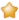19,519June 2005
 [Python] Tic-Tac-Toe Jun 23, 2009 15:42:39 GMT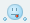You would. Making simple games are always fun though.Anyways, I think this is more... Open Source than a tutorial. Wrong board perhaps?Michael*Has a custom title*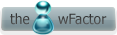1,462October 2007
 [Python] Tic-Tac-Toe Jun 23, 2009 16:05:23 GMT   I thought OS too!Eric1,442November 2005
 [Python] Tic-Tac-Toe Jun 23, 2009 16:24:52 GMT   Perhaps would be correct. Don't ask, it was ridiculously early in the morning.BTW, cookie points if you can work out how my choose_move algorithm works. Last Edit: Jun 23, 2009 16:29:07 GMT by Eric

new